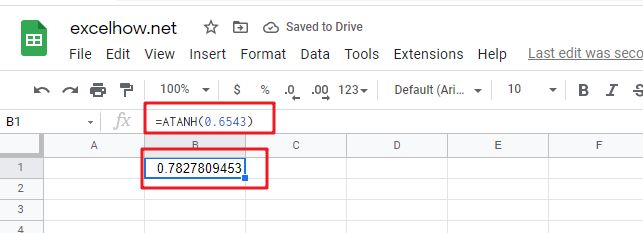This post will guide you how to use Google Sheets ATANH function with syntax and examples.

## Description

The Google Sheets ATANH function returns the inverse hyperbolic tangent of a given number. So you can use the ATANH function to calculate the inverse hyperbolic tangent for a number. The returned result is a numeric value.

The ATANH function is a build-in function in Google Sheets and it is categorized as a MATH function.

## Syntax

The syntax of the ATANH function is as below:

= ATANH (number)

Where the ATANH function arguments is:

• number -This is a required argument. A real number between 1 and -1, but it should exclude the values of -1 and 1.

Note:

• If the number argument is greater than or equal to 1, the ATANH function will return #NUM! Error. If the number argument is less than or equal to -1, the ATANH function will return #NUM! Error.
• If the supplied number is not a numeric value, the ATANH function will get the #VALUE！ Error。

## Google Sheets ATANH Function Examples

The below examples will show you how to use google sheets ATANH Function to return the arctangent of x and y coordinates.

1# get inverse hyperbolic tangent of 0.6543, enter the following formula in Cell B1.

=ATANH(0.6543)

The returned result is 0.782781.